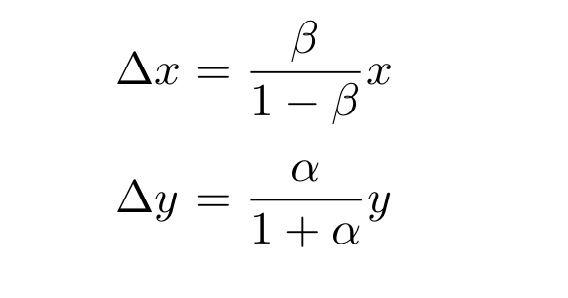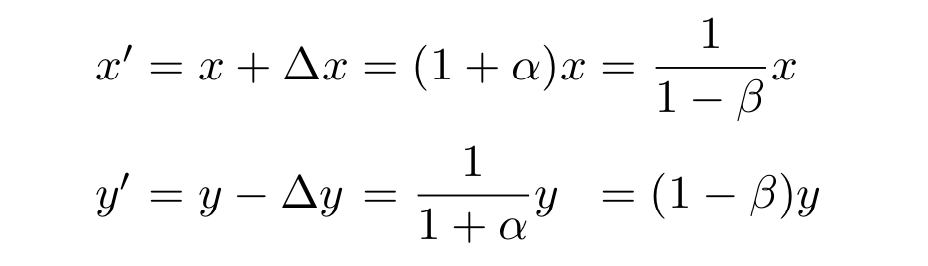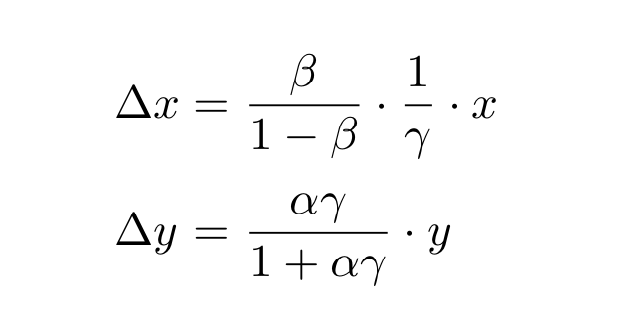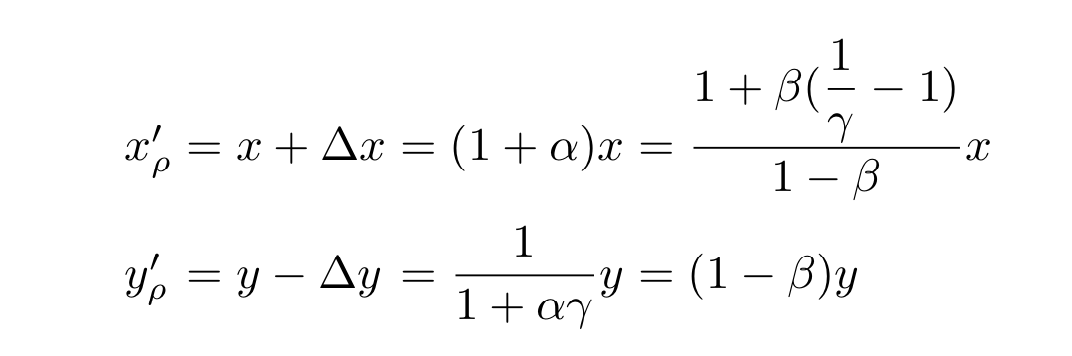### 区块链中的数学-Uniwap自动化做市商核心算法解析

• blocksight
• 更新于 2020-09-21 10:25
• 阅读 9154

Uniswap协议采用的是常量乘积做市商模型，又称为“恒定乘积做市商模型“。

## 常量乘积做市商模型

Uniswap协议采用的是常量乘积做市商模型，又称为“恒定乘积做市商模型“。数学公式表示为 x * y = k, 通过确定某一时刻x,y的兑换比例，来实现x和y之间的自动交易。所有规则定义在合约中，由区块链系统（如以太坊）自动执行，省去第三方做市商参与， 有时也称为：x-y-k自动做市商模型

### x-y-k模型

x-y-k模型中，x, y分别一个交易对（Pair/Pool）中某一时间两种代币的余额，x,y 对应值两种不同的代币，在目前uniswap版本中，目前只支持ERC20代币。

$x' = x + ∆x = (1 + \alpha ) x$ $y' = y - ∆y = (1 - \beta) y$

$\alpha= ∆x / x \ ,\ \beta = ∆y / y$

$1+\alpha=\frac{1}{1-\beta }$## 添加费用机制的x-y-k模型

$x' = x + ∆x = (1 + \alpha （1 - ρ ）) * x$

$y' = y - ∆y = (1 -\beta ) * y$

$\Delta x =\frac{\beta }{1-\beta } \frac{1}{1-\rho } x$

$\Delta y=\frac{\alpha (1-\rho)}{1+\alpha(1-\rho )} * y$$1+\beta(\frac{1}{\gamma }-1)$

uniswap的流动性生成体现出乘积k的作用， 使用$\sqrt{k}$ 作为流动性增长的依据，下一篇会详细说明。

## 小结

uniswap 做市商模型简单而优雅，运行近两年没有出现大的安全问题。从本节的分析中可以看到，并无高等数学积分，离散，分布等内容，高中数学水平就能理解，这也印证了简洁的力量。